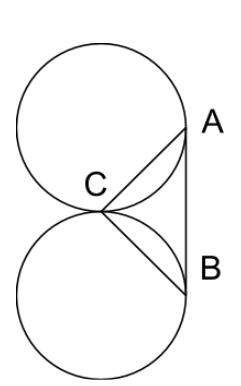# Kissing Circles For Valentines Day

Geometry Level 2Two circles of with equal radii are tangent to each other at point $C$. Line segment $AB$ is tangent to both circles, where $A$ and $B$ are the points of tangency.

Let the area of $\triangle ABC$ be $x$ and let the combined area of the circles be $y$. What is $\frac{y}{x}$ rounded to 4 decimal places?

×